Levels »

# Never Stop Firing

### The Pyramid, Elevation: 2.7m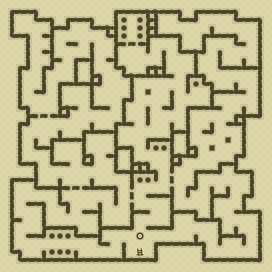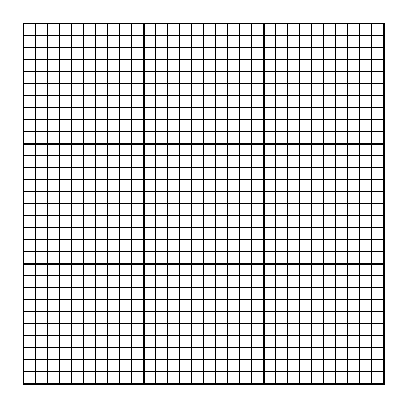### Items

•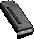8 Walther P4 Magazines (61 rounds) (4, 6) x8 - (22, 6) x5 - (7, 10) x8 - (26, 11) x8 - (21, 14) x8 - (6, 17) x8 - (10, 17) x8 - (26, 22) x8
•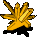Yellow Crystal (15, 1)

### Monsters

•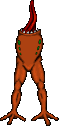49 Headless (Random rate: 3) (4, 2) - (9, 3) - (4, 4) - (23, 4) - (23, 4) - (9, 5) - (12, 5) - (16, 5) - (4, 6) - (14, 6) - (30, 6) - (16, 7) - (20, 9) - (27, 9) - (8, 11) - (11, 11) - (17, 11) - (19, 11) - (23, 11) - (5, 12) - (30, 12) - (15, 13) - (21, 14) - (24, 14) - (7, 15) - (27, 15) - (4, 16) - (14, 16) - (12, 17) - (7, 18) - (18, 19) - (13, 21) - (18, 22) - (26, 22) - (3, 23) - (7, 23) - (8, 24) - (21, 24) - (27, 24) - (18, 25) - (24, 25) - (19, 26) - (21, 26) - (3, 27) - (27, 27) - (24, 28) - (5, 29) - (27, 29) - (3, 30)
•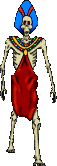3 Zombies (2, 2) - (21, 2) - (15, 6)

### Locations

• Unknown 6 trigger (15, 1)
• Left chain (2, 2)
• Right chain (20, 2)
• 4 Door openers (4, 12) - (8, 21) - (21, 21) - (14, 23)
• Save rune(16, 28)